SLAS719E August   2010  – June 2015

PRODUCTION DATA.

1. Features
2. Applications
3. Description
4. Revision History
5. Device Comparison Table
6. Pin Configuration and Functions
7. Specifications
8. Detailed Description
1. 8.1 Overview
2. 8.2 Functional Block Diagram
3. 8.3 Feature Description
1. 8.3.1 Digital-to-Analog Converter (DAC)
2. 8.3.2 Internal Reference
3. 8.3.3 Power-On Reset
4. 8.4 Device Functional Modes
5. 8.5 Programming
9. Application and Implementation
1. 9.1 Application Information
1. 9.1.1 DAC Internal Reference
2. 9.1.2 DAC Noise Performance
2. 9.2 Typical Applications
3. 9.3 System Examples
10. 10Power Supply Recommendations
11. 11Layout
12. 12Device and Documentation Support
13. 13Mechanical, Packaging, and Orderable Information

• DGS|10
• DSC|10
• DSC|10

## 7 Specifications

### 7.1 Absolute Maximum Ratings(1)

Over operating ambient temperature range (unless otherwise noted).
MIN MAX UNIT
AVDD to GND –0.3 6 V
CLR, DIN, LDAC, SCLK and SYNC input voltage to GND –0.3 AVDD + 0.3 V
VOUT[A, B] to GND –0.3 AVDD + 0.3 V
VREFIN/VREFOUT to GND –0.3 AVDD + 0.3 V
Operating temperature range –40 125 °C
Junction temperature, TJ 150 °C
Storage temperature, Tstg –65 150 °C
(1) Stresses beyond those listed under Absolute Maximum Ratings may cause permanent damage to the device. These are stress ratings only, and do not imply functional operation of the device at these or any other conditions beyond those indicated under Recommended Operating Conditions. Exposure to absolute-maximum-rated conditions for extended periods may affect device reliability.

### 7.2 ESD Ratings

VALUE UNIT
V(ESD) Electrostatic discharge Human-body model (HBM), per ANSI/ESDA/JEDEC JS-001(1) ±1000 V
Charged-device model (CDM), per JEDEC specification JESD22-C101(2) ±500
(1) JEDEC document JEP155 states that 500-V HBM allows safe manufacturing with a standard ESD control process.
(2) JEDEC document JEP157 states that 250-V CDM allows safe manufacturing with a standard ESD control process.

### 7.3 Recommended Operating Conditions

over operating ambient temperature range (unless otherwise noted)
MIN NOM MAX UNIT
POWER SUPPLY
Supply voltage AVDD to GND 2.7 5.5 V
DIGITAL INPUTS
Digital input voltage CLR, DIN, LDAC, SCLK and SYNC 0 AVDD V
REFERENCE INPUT
VREFIN Reference input voltage 0 AVDD V
TEMPERATURE RANGE
TA Operating ambient temperature –40 125 °C

### 7.4 Thermal Information

THERMAL METRIC DAC756x, DAC816x, DAC856x UNIT
DSC (WSON) DGS (VSSOP)
10 PINS 10 PINS
RθJA Junction-to-ambient thermal resistance 62.8 173.8 °C/W
RθJC(top) Junction-to-case (top) thermal resistance 44.3 48.5 °C/W
RθJB Junction-to-board thermal resistance 26.5 79.9 °C/W
ψJT Junction-to-top characterization parameter 0.4 1.7 °C/W
ψJB Junction-to-board characterization parameter 25.5 68.4 °C/W
RθJC(bot) Junction-to-case (bottom) thermal resistance 46.2 N/A °C/W

### 7.5 Electrical Characteristics

At AVDD = 2.7 V to 5.5 V and TA = –40°C to 125°C (unless otherwise noted).
PARAMETER TEST CONDITIONS MIN TYP MAX UNIT
STATIC PERFORMANCE(1)
DAC856x Resolution 16 Bits
Relative accuracy Using line passing through codes 512 and 65,024 ±4 ±12 LSB
Differential nonlinearity 16-bit monotonic ±0.2 ±1 LSB
DAC816x Resolution 14 Bits
Relative accuracy Using line passing through codes 128 and 16,256 ±1 ±3 LSB
Differential nonlinearity 14-bit monotonic ±0.1 ±0.5 LSB
DAC756x Resolution 12 Bits
Relative accuracy Using line passing through codes 32 and 4,064 ±0.3 ±0.75 LSB
Differential nonlinearity 12-bit monotonic ±0.05 ±0.25 LSB
Offset error Extrapolated from two-point line(1), unloaded ±1 ±4 mV
Offset error drift ±2 µV/°C
Full-scale error DAC register loaded with all 1s ±0.03 ±0.2 % FSR
Zero-code error DAC register loaded with all 0s 1 4 mV
Zero-code error drift ±2 µV/°C
Gain error Extrapolated from two-point line(1), unloaded ±0.01 ±0.15 % FSR
Gain temperature coefficient ±1 ppm FSR/°C
OUTPUT CHARACTERISTICS(2)
Output voltage range 0 AVDD V
Output voltage settling time(3) DACs unloaded 7 µs
RL = 1 MΩ 10
Slew rate Measured between 20%–80% of a full-scale transition 0.75 V/µs
Capacitive load stability RL = ∞ 1 nF
RL = 2 kΩ 3
Code-change glitch impulse 1-LSB change around major carry 0.1 nV-s
Digital feedthrough SCLK toggling, SYNC high 0.1 nV-s
Power-on glitch impulse RL = 2 kΩ, CL = 470 pF, AVDD = 5.5 V 40 mV
Channel-to-channel dc crosstalk Full-scale swing on adjacent channel,
External reference
5 µV
Internal reference
15
DC output impedance At mid-scale input 5 Ω
Short-circuit current DAC outputs at full-scale, DAC outputs shorted to GND 40 mA
Power-up time, including settling time Coming out of power-down mode 50 µs
AC PERFORMANCE(2)
DAC output noise density TA = 25°C, at mid-scale input, fOUT = 1 kHz 90 nV/√Hz
DAC output noise TA = 25°C, at mid-scale input, 0.1 Hz to 10 Hz 2.6 µVPP
LOGIC INPUTS(2)
Input-pin leakage current –1 ±0.1 1 µA
Logic input LOW voltage VIL 0 0.8 V
Logic input HIGH voltage VIH 0.7 × AVDD AVDD V
Pin capacitance 3 pF
REFERENCE
External reference current External VREF = 2.5 V (when internal reference is disabled), all channels active using gain = 1 15 µA
Reference input impedance Internal reference disabled, gain = 1 170
Internal reference disabled, gain = 2 85
REFERENCE OUTPUT
Output voltage TA = 25°C 2.495 2.5 2.505 V
Initial accuracy TA = 25°C –5 ±0.1 5 mV
Output-voltage temperature drift 4 10 ppm/°C
Output-voltage noise f = 0.1 Hz to 10 Hz 12 µVPP
Output-voltage noise density (high-frequency noise) TA = 25°C, f = 1 kHz, CL = 0 µF 250 nV/√Hz
TA = 25°C, f = 1 MHz, CL = 0 µF 30
TA = 25°C, f = 1 MHz, CL = 4.7 µF 10
Load regulation, sourcing(4) TA = 25°C 20 µV/mA
Load regulation, sinking(4) TA = 25°C 185 µV/mA
Line regulation TA = 25°C 50 µV/V
Long-term stability or drift (aging)(4) TA = 25°C, time = 0 to 1900 hours 100 ppm
Thermal hysteresis(4) First cycle 200 ppm
POWER REQUIREMENTS(5)
Power supply current (IDD) AVDD = 3.6 V to 5.5 V, normal mode, internal reference off 0.25 0.5 mA
AVDD = 3.6 V to 5.5 V, normal mode, internal reference on 0.9 1.6
AVDD = 3.6 V to 5.5 V, power-down modes(6) 0.55 2 µA
AVDD = 3.6 V to 5.5 V, power-down modes 0.55 4
AVDD = 2.7 V to 3.6 V, normal mode, internal reference off 0.2 0.4 mA
AVDD = 2.7 V to 3.6 V, normal mode, internal reference on 0.73 1.4
AVDD = 2.7 V to 3.6 V, power-down modes(6) 0.35 2 µA
AVDD = 2.7 V to 3.6 V, power-down modes 0.35 3
Power dissipation AVDD = 3.6 V to 5.5 V, normal mode, internal reference off 0.9 2.75 mW
AVDD = 3.6 V to 5.5 V, normal mode, internal reference on 3.2 8.8
AVDD = 3.6 V to 5.5 V, power-down modes(6) 2 11 µW
AVDD = 3.6 V to 5.5 V, power-down modes 2 22
AVDD = 2.7 V to 3.6 V, normal mode, internal reference off 0.54 1.44 mW
AVDD = 2.7 V to 3.6 V, normal mode, internal reference on 1.97 5
AVDD = 2.7 V to 3.6 V, power-down modes(6) 0.95 7.2 µW
AVDD = 2.7 V to 3.6 V, power-down modes 0.95 10.8
(1) 16-bit: codes 512 and 65,024; 14-bit: codes 128 and 16,256; 12-bit: codes 32 and 4,064
(2) Specification based on design or characterization. Not production tested
(3) Transition time between 1 / 4 scale and 3 / 4 scale, including settling to within ±0.024% FSR
(4) See the Application Information section of this data sheet.
(5) Input code = mid-scale, no load, VINH = AVDD, and VINL = GND
(6) TA = –40°C to 105°C

### 7.6 Timing Requirements(1)(2)

At AVDD = 2.7 V to 5.5 V and over –40°C to 125°C (unless otherwise noted).
DAC756x, DAC816x, DAC856x UNIT
MIN TYP MAX
f(SCLK) Serial clock frequency 50 MHz
t(1) SCLK falling edge to SYNC falling edge (for successful write operation) 10 ns
t(2) SCLK cycle time 20 ns
t(3) SYNC rising edge to 23rd SCLK falling edge (for successful SYNC interrupt) 13 ns
t(4) Minimum SYNC HIGH time 80 ns
t(5) SYNC to SCLK falling edge setup time 13 ns
t(6) SCLK LOW time 8 ns
t(7) SCLK HIGH time 8 ns
t(8) SCLK falling edge to SYNC rising edge 10 ns
t(9) Data setup time 6 ns
t(10) Data hold time 5 ns
t(11) SCLK falling edge to LDAC falling edge for asynchronous LDAC update mode 5 ns
t(12) LDAC pulse duration, LOW time 10 ns
t(13) CLR pulse duration, LOW time 80 ns
t(14) CLR falling edge to start of VOUT transition 100 ns
(1) All input signals are specified with tr = tf = 3 ns (10% to 90% of AVDD) and timed from a voltage level of (VIL + VIH) / 2.
(2) See the Serial Write Operation timing diagram (Figure 1).1. Asynchronous LDAC update mode. For more information, see the LDAC Functionality section.
2. Synchronous LDAC update mode; LDAC remains low. For more information, see the LDAC Functionality section.
Figure 1. Serial Write Operation

### Table 1. Typical Characteristics: Internal Reference Performance

MEASUREMENT POWER-SUPPLY VOLTAGE FIGURE NUMBER
Internal Reference Voltage vs Temperature 5.5 V Figure 2
Internal Reference Voltage Temperature Drift Histogram Figure 3
Internal Reference Voltage vs Load Current Figure 4
Internal Reference Voltage vs Time Figure 5
Internal Reference Noise Density vs Frequency Figure 6
Internal Reference Voltage vs Supply Voltage 2.7 V–5.5 V Figure 7

### Table 2. Typical Characteristics: DAC Static Performance

MEASUREMENT POWER-SUPPLY VOLTAGE FIGURE NUMBER
FULL-SCALE, GAIN, OFFSET AND ZERO-CODE ERRORS
Full-Scale Error vs Temperature 5.5 V Figure 16
Gain Error vs Temperature Figure 17
Offset Error vs Temperature Figure 18
Zero-Code Error vs Temperature Figure 19
Full-Scale Error vs Temperature 2.7 V Figure 63
Gain Error vs Temperature Figure 64
Offset Error vs Temperature Figure 65
Zero-Code Error vs Temperature Figure 66
DAC Output Voltage vs Load Current 5.5 V Figure 30
2.7 V Figure 74
DIFFERENTIAL NONLINEARITY ERROR
Differential Linearity Error vs Digital Input Code T = –40°C 5.5 V Figure 9
T = 25°C Figure 11
T = 125°C Figure 13
Differential Linearity Error vs Temperature Figure 15
Differential Linearity Error vs Digital Input Code T = –40°C 2.7 V Figure 56
T = 25°C Figure 58
T = 125°C Figure 60
Differential Linearity Error vs Temperature Figure 62
INTEGRAL NONLINEARITY ERROR (RELATIVE ACCURACY)
Linearity Error vs Digital Input Code T = –40°C 5.5 V Figure 8
T = 25°C Figure 10
T = 125°C Figure 12
Linearity Error vs Temperature Figure 14
Linearity Error vs Digital Input Code T = –40°C 2.7 V Figure 55
T = 25°C Figure 57
T = 125°C Figure 59
Linearity Error vs Temperature Figure 61
POWER-DOWN CURRENT
Power-Down Current vs Temperature 5.5 V Figure 28
Power-Down Current vs Power-Supply Voltage 2.7 V – 5.5 V Figure 29
Power-Down Current vs Temperature 2.7 V Figure 73
POWER-SUPPLY CURRENT
Power-Supply Current vs Temperature External VREF 5.5 V Figure 20
Internal VREF Figure 21
Power-Supply Current vs Digital Input Code External VREF Figure 22
Internal VREF Figure 23
Power-Supply Current Histogram External VREF Figure 24
Internal VREF Figure 25
Power-Supply Current vs Power-Supply Voltage External VREF 2.7 V – 5.5 V Figure 26
Internal VREF Figure 27
Power-Supply Current vs Temperature External VREF 3.6 V Figure 49
Internal VREF Figure 50
Power-Supply Current vs Digital Input Code External VREF Figure 51
Internal VREF Figure 52
Power-Supply Current Histogram External VREF Figure 53
Internal VREF Figure 54
Power-Supply Current vs Temperature External VREF 2.7 V Figure 67
Internal VREF Figure 68
Power-Supply Current vs Digital Input Code External VREF Figure 69
Internal VREF Figure 70
Power-Supply Current Histogram External VREF Figure 71
Internal VREF Figure 72

### Table 3. Typical Characteristics: DAC Dynamic Performance

MEASUREMENT POWER-SUPPLY VOLTAGE FIGURE NUMBER
CHANNEL-TO-CHANNEL CROSSTALK
Channel-to-Channel Crosstalk 5-V Rising Edge 5.5 V Figure 43
5-V Falling Edge Figure 44
CLOCK FEEDTHROUGH
Clock Feedthrough 500 kHz, Midscale 5.5 V Figure 48
2.7 V Figure 87
GLITCH IMPULSE
Glitch Impulse, 1-LSB Step Rising Edge, Code 7FFFh to 8000h 5.5 V Figure 37
Falling Edge, Code 8000h to 7FFFh Figure 38
Glitch Impulse, 4-LSB Step Rising Edge, Code 7FFCh to 8000h Figure 39
Falling Edge, Code 8000h to 7FFCh Figure 40
Glitch Impulse, 16-LSB Step Rising Edge, Code 7FF0h to 8000h Figure 41
Falling Edge, Code 8000h to 7FF0h Figure 42
Glitch Impulse, 1-LSB Step Rising Edge, Code 7FFFh to 8000h 2.7 V Figure 79
Falling Edge, Code 8000h to 7FFFh Figure 80
Glitch Impulse, 4-LSB Step Rising Edge, Code 7FFCh to 8000h Figure 81
Falling Edge, Code 8000h to 7FFCh Figure 82
Glitch Impulse, 16-LSB Step Rising Edge, Code 7FF0h to 8000h Figure 83
Falling Edge, Code 8000h to 7FF0h Figure 84
NOISE
DAC Output Noise Density vs Frequency External VREF 5.5 V Figure 45
Internal VREF Figure 46
DAC Output Noise 0.1 Hz to 10 Hz External VREF Figure 47
POWER-ON GLITCH
Power-On Glitch Reset to Zero Scale 5.5 V Figure 35
Reset to Midscale Figure 36
Reset to Zero Scale 2.7 V Figure 85
Reset to Midscale Figure 86
SETTLING TIME
Full-Scale Settling Time Rising Edge, Code 0h to FFFFh 5.5 V Figure 31
Falling Edge, Code FFFFh to 0h Figure 32
Half-Scale Settling Time Rising Edge, Code 4000h to C000h Figure 33
Falling Edge, Code C000h to 4000h Figure 34
Full-Scale Settling Time Rising Edge, Code 0h to FFFFh 2.7 V Figure 75
Falling Edge, Code FFFFh to 0h Figure 76
Half-Scale Settling Time Rising Edge, Code 4000h to C000h Figure 77
Falling Edge, Code C000h to 4000h Figure 78

#### 7.7.1 Typical Characteristics: Internal Reference

At TA = 25°C, AVDD = 5.5 V, gain = 2, and VREFOUT unloaded, unless otherwise noted.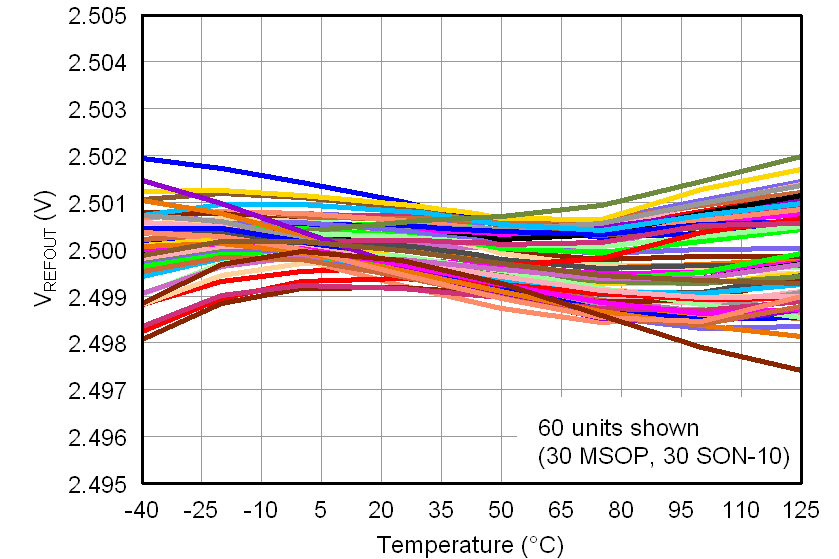Figure 2. Internal Reference Voltage vs Temperature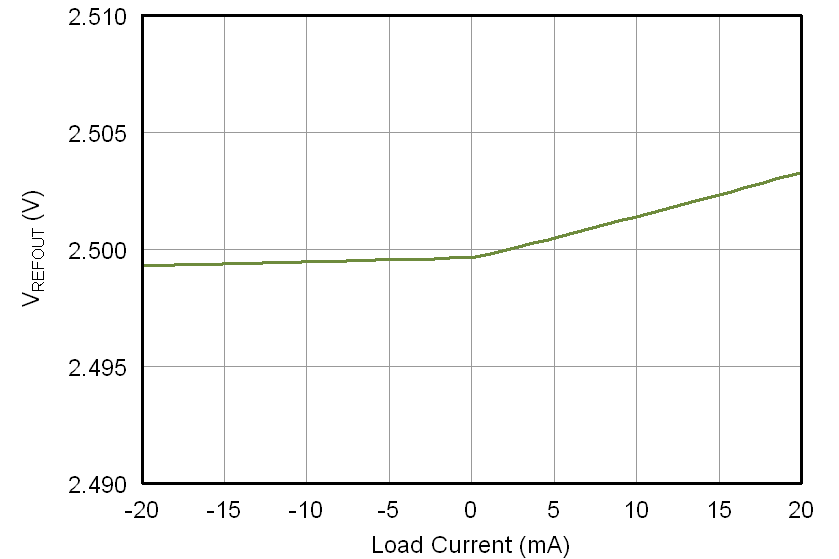Figure 4. Internal Reference Voltage vs Load CurrentFigure 6. Internal Reference Noise Density vs FrequencyFigure 3. Internal Reference Voltage, Temperature Drift HistogramFigure 5. Internal Reference Voltage vs TimeFigure 7. Internal Reference Voltage vs Supply Voltage

#### 7.7.2 Typical Characteristics: DAC at AVDD = 5.5 V

At TA = 25°C, 5-V external reference used, gain = 1 and DAC output not loaded, unless otherwise noted.Figure 8. Linearity Error vs Digital Input Code (–40°C)Figure 10. Linearity Error vs Digital Input Code (25°C)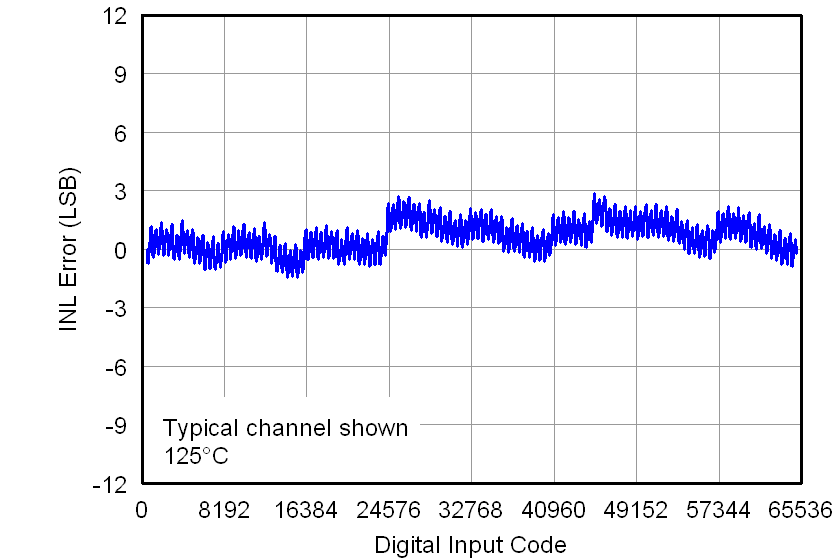Figure 12. Linearity Error vs Digital Input Code (125°C)Figure 14. Linearity Error vs Temperature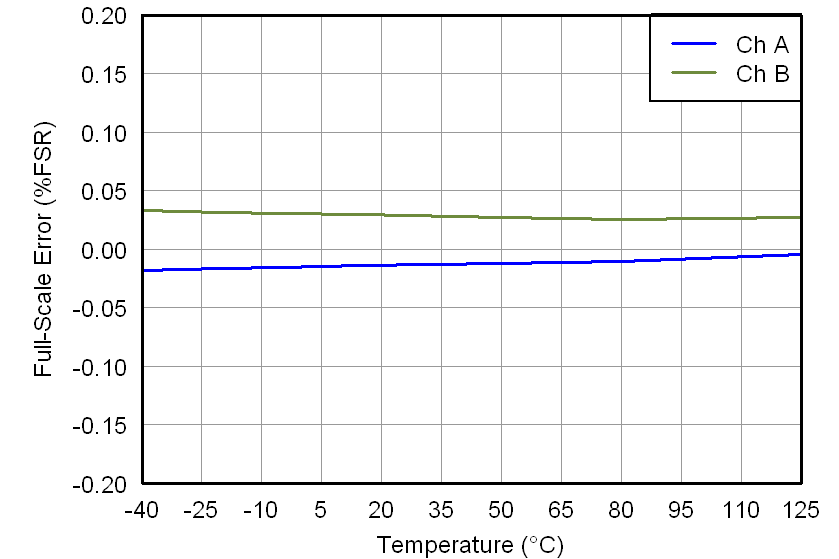Figure 16. Full-Scale Error vs TemperatureFigure 18. Offset Error vs TemperatureFigure 20. Power-Supply Current vs TemperatureFigure 22. Power-Supply Current vs Digital Input CodeFigure 24. Power-Supply Current HistogramFigure 26. Power-Supply Current vs Power-Supply VoltageFigure 28. Power-Down Current vs TemperatureFigure 30. DAC Output Voltage vs Load Current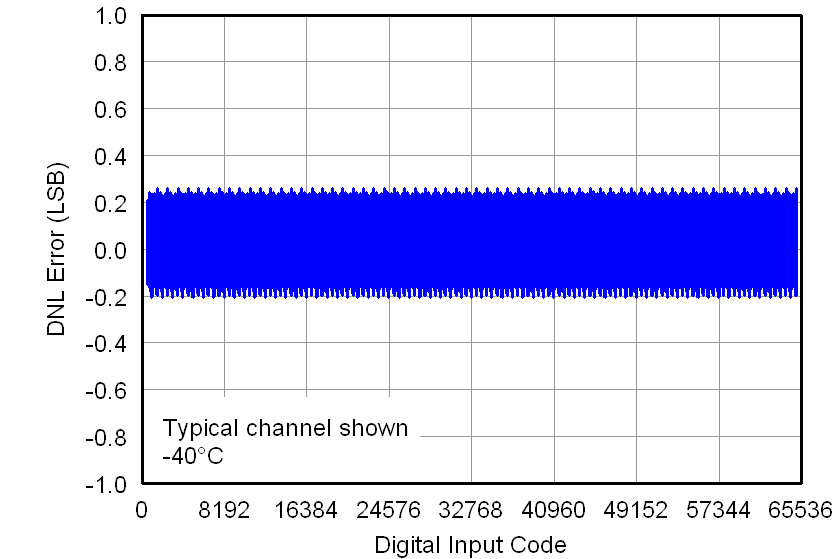Figure 9. Differential Linearity Error vs Digital Input Code (–40°C)Figure 11. Differential Linearity Error vs Digital Input Code (25°C)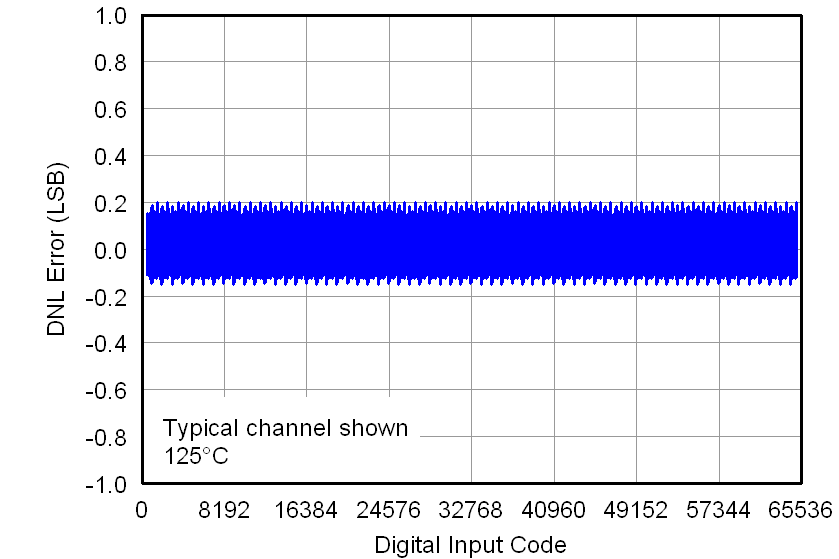Figure 13. Differential Linearity Error vs Digital Input Code (125°C)Figure 15. Differential Linearity Error vs TemperatureFigure 17. Gain Error vs TemperatureFigure 19. Zero-Code Error vs TemperatureFigure 21. Power-Supply Current vs TemperatureFigure 23. Power-Supply Current vs Digital Input CodeFigure 25. Power-Supply Current HistogramFigure 27. Power-Supply Current vs Power-Supply VoltageFigure 29. Power-Down Current vs Power-Supply VoltageFigure 31. Full-Scale Settling Time, Rising EdgeFigure 33. Half-Scale Settling Time, Rising Edge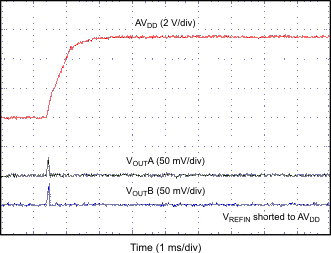Figure 35. Power-On Glitch, Reset to Zero ScaleFigure 37. Glitch Impulse, Rising Edge, 1-LSB Step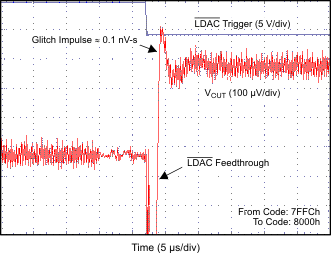Figure 39. Glitch Impulse, Rising Edge, 4-LSB StepFigure 41. Glitch Impulse, Rising Edge, 16-LSB Step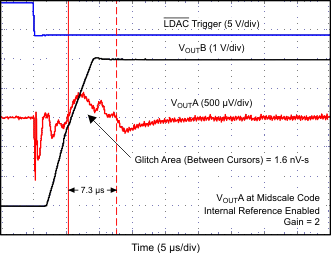Figure 43. Channel-to-Channel Crosstalk, 5-V Rising Edge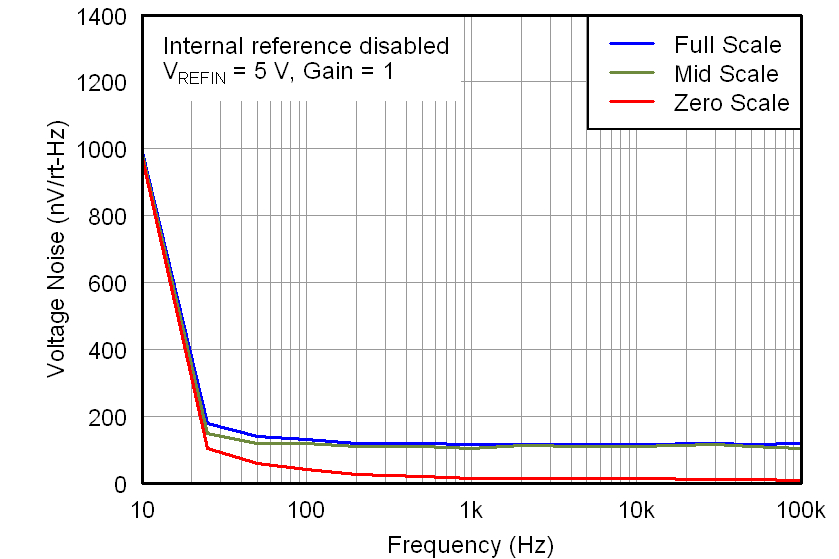Figure 45. DAC Output Noise Density vs FrequencyFigure 47. DAC Output Noise, 0.1 Hz to 10 Hz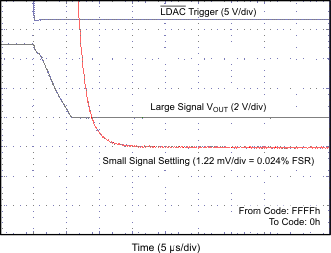Figure 32. Full-Scale Settling Time, Falling EdgeFigure 34. Half-Scale Settling Time, Falling Edge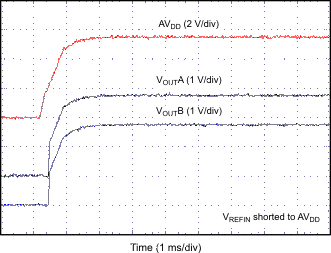Figure 36. Power-On Glitch, Reset to MidscaleFigure 38. Glitch Impulse, Falling Edge, 1-LSB StepFigure 40. Glitch Impulse, Falling Edge, 4-LSB StepFigure 42. Glitch Impulse, Falling Edge, 16-LSB Step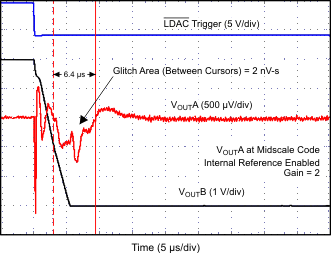Figure 44. Channel-to-Channel Crosstalk, 5-V Falling Edge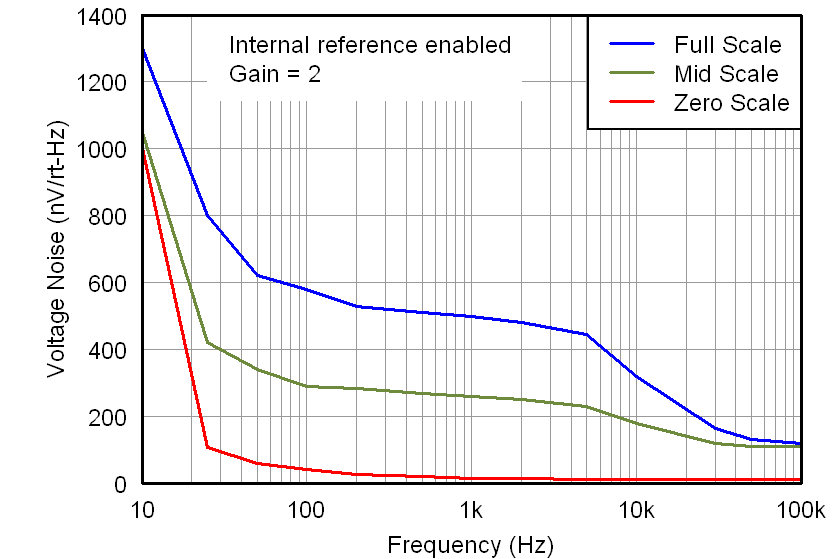Figure 46. DAC Output Noise Density vs Frequency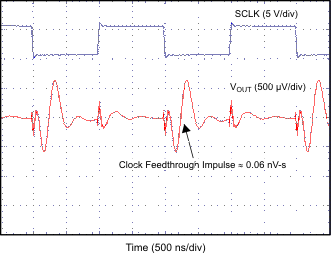Figure 48. Clock Feedthrough, 500 kHz, Midscale

#### 7.7.3 Typical Characteristics: DAC at AVDD = 3.6 V

At TA = 25°C, 3.3-V external reference used, gain = 1 and DAC output not loaded, unless otherwise noted.Figure 49. Power-Supply Current vs TemperatureFigure 51. Power-Supply Current vs Digital Input Code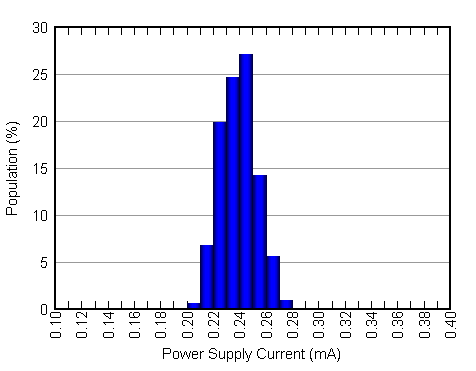Figure 53. Power-Supply Current Histogram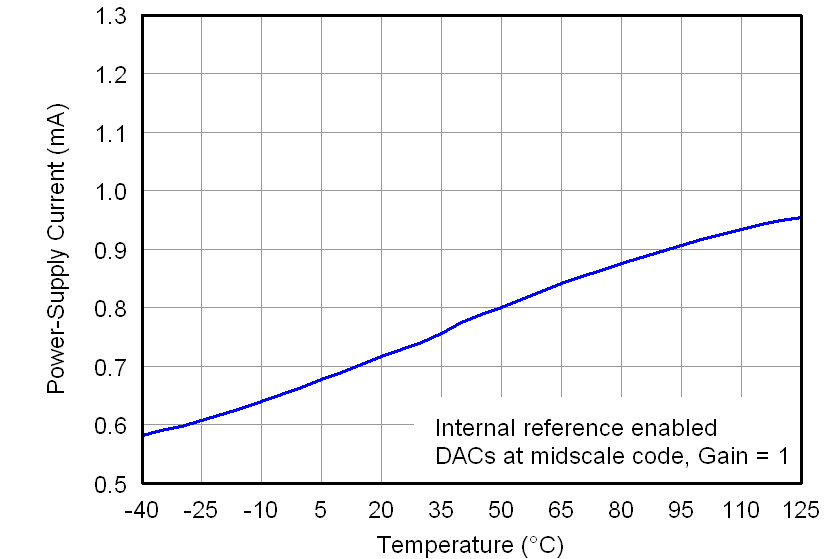Figure 50. Power-Supply Current vs TemperatureFigure 52. Power-Supply Current vs Digital Input CodeFigure 54. Power-Supply Current Histogram

#### 7.7.4 Typical Characteristics: DAC at AVDD = 2.7 V

At TA = 25°C, 2.5-V external reference used, gain = 1, and DAC output not loaded, unless otherwise noted.Figure 55. Linearity Error vs Digital Input Code (–40°C)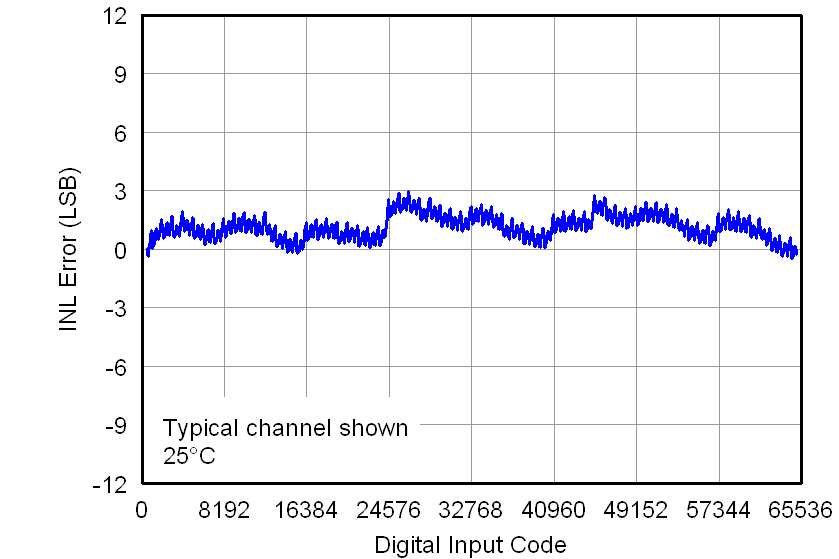Figure 57. Linearity Error vs Digital Input Code (25°C)Figure 59. Linearity Error vs Digital Input Code (125°C)Figure 61. Linearity Error vs TemperatureFigure 63. Full-Scale Error vs Temperature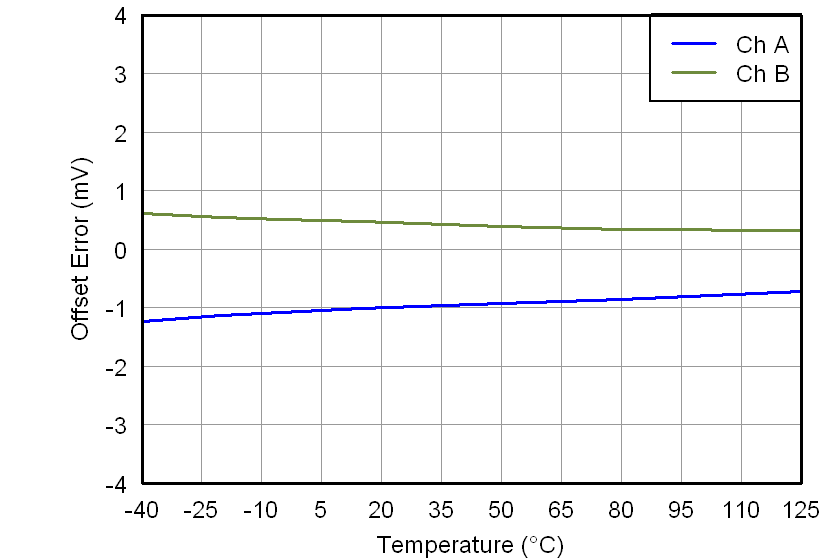Figure 65. Offset Error vs TemperatureFigure 67. Power-Supply Current vs Temperature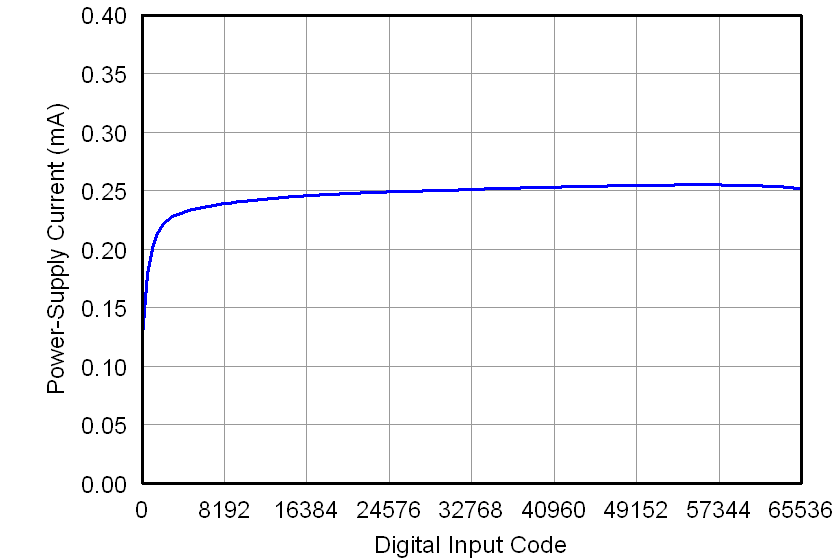Figure 69. Power-Supply Current vs Digital Input Code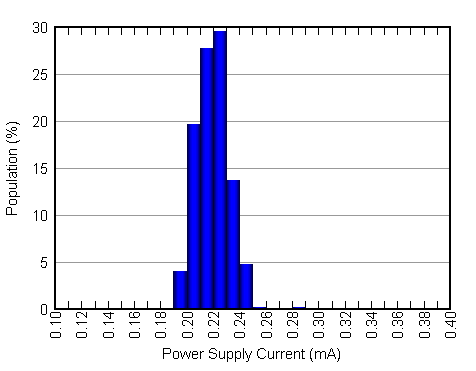Figure 71. Power-Supply Current HistogramFigure 73. Power-Down Current vs TemperatureFigure 75. Full-Scale Settling Time, Rising EdgeFigure 77. Half-Scale Settling Time, Rising EdgeFigure 79. Glitch Impulse, Rising Edge, 1-LSB StepFigure 81. Glitch Impulse, Rising Edge, 4-LSB StepFigure 83. Glitch Impulse, Rising Edge, 16-LSB StepFigure 85. Power-On Glitch, Reset to Zero ScaleFigure 87. Clock Feedthrough, 500 kHz, MidscaleFigure 56. Differential Linearity Error vs Digital Input Code (–40°C)Figure 58. Differential Linearity Error vs Digital Input Code (25°C)Figure 60. Differential Linearity Error vs Digital Input Code (125°C)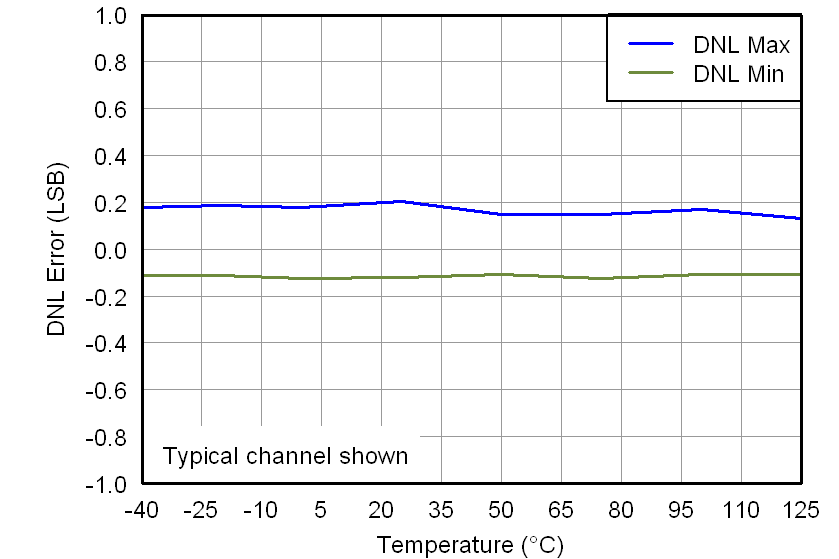Figure 62. Differential Linearity Error vs TemperatureFigure 64. Gain Error vs TemperatureFigure 66. Zero-Code Error vs Temperature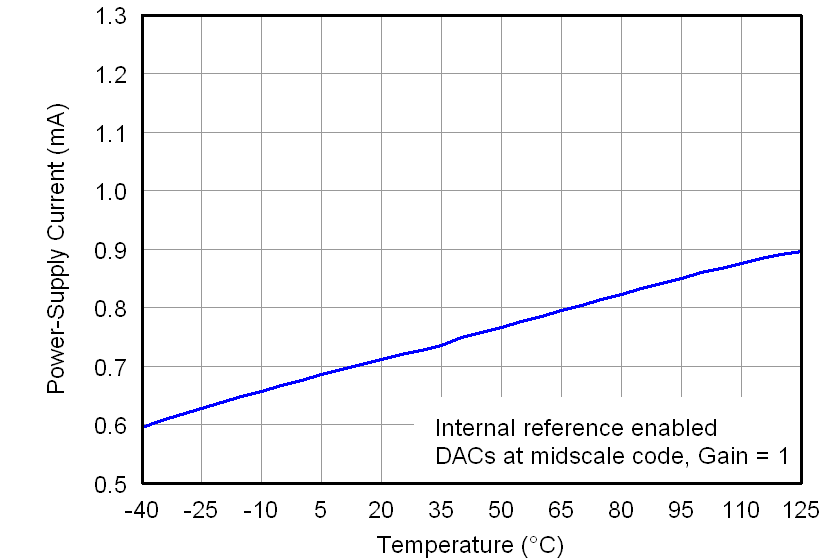Figure 68. Power-Supply Current vs TemperatureFigure 70. Power-Supply Current vs Digital Input Code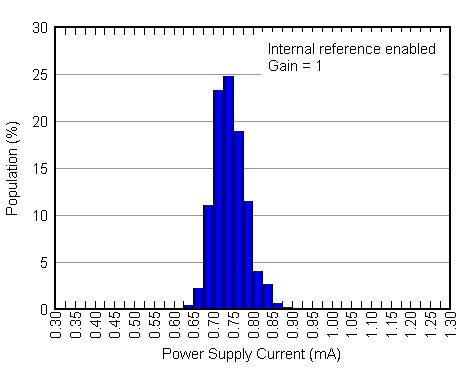Figure 72. Power-Supply Current HistogramFigure 74. DAC Output Voltage vs Load Current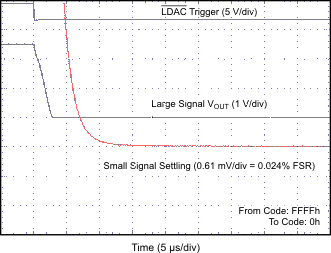Figure 76. Full-Scale Settling Time, Falling EdgeFigure 78. Half-Scale Settling Time, Falling EdgeFigure 80. Glitch Impulse, Falling Edge, 1-LSB Step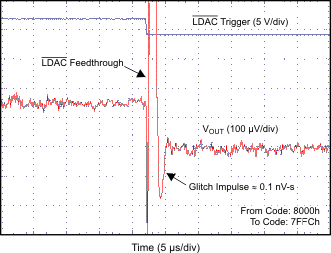Figure 82. Glitch Impulse, Falling Edge, 4-LSB Step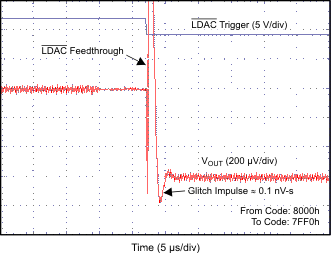Figure 84. Glitch Impulse, Falling Edge, 16-LSB StepFigure 86. Power-On Glitch, Reset to Midscale